# 22LL

Case naming: XYZ
X is the sticker to which UBR goes. Y and Z are two cycled edge stickers
(Letters taken from Speffz notation)
(?) after an alg means there might be something better. If you have something, send me a message.

A set
AAC AAI AAB AAMy R U R' U' R' F R2 U' R' U' R U R' F' y' y R2 B' R' B R' F' U' F R U R' U' y' y R U R' F' R U R' U' R' F R2 U' R' U' y' r' U2 R U2 R' F R U R' U' R' F' R2 U' M'
AAD AAE ABD ABE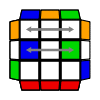y R' U2 R U R' U2' L U' R U L' y' l U2 L' U2 L F' L' U' L U L F L2 U M' y R' U' F' R U R' U' R' F R2 U' R' U' R U R' U R y' y R U2 R' U2 R' F R U R U2 R' U' R U R' F' y'
ABC ABI ACD ACER' U2 R U2 R' F R U R' U' R' F' R2 U' L F R F' L' U R U' F U R U' F' U R2 U' (?) L U2 L' U2 L F' L' U' L U L F L2 U M' U R' U2 R U R' U2' L U' R U L' U' M (?)
E set
EAC EAI EAB EAMy2 r U' r U2 R' F R U2 r2 F y2 F U R' U' R D' R2 U R' U' R2 D F' r U R2 F R F' R U2 r' U r U r' y' R U2 R' U' F' U R U R' U' R' F R2 U' R' U2 y
EAD EAE EBD EBEy2 L' U R' U' R L U2 R' U' R U2 B' U R' U' R' F R2 U' R' U' R U R' F' R B r U r' x R2' U2' R U R' U2' L U' R U R U' r' y F R' F R F' R U' R' F' U F R U R' F' y'
EBC EBI ECD ECER U2 R' U' R' F R2 U' R' U' R U R' F' R U' R' R U R' U R U2 B' R' U' R U B U' R' U2 y' R U R D R' U' R D' R' U2 R' U' R U' R' U' y y2 R' U' R U' R' U2 F R U R' U' F' U R U2
R set
RAC RAI RAB RAML U R' U L' U2 R U' R' L U L' U2 R U' R' U R' F' U2 F U' R B' R B R2 U' R2 y' F2 R U' R' U' R U R' F' R U R' U' R' F R F2 y r' R U R U' L U L' U R' U' r B2 R B2 R2'
RAD RAE RBD RBE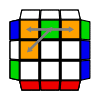R' F R U' R' U' R U R' F' R U R' U' R' F R F' R l L' U' L' U R' U' R U' L U l' B2 L' B2 L2 R U' R' U R U' L U L' U B2 R B2 R2' y R L' U R' U' L F2 L F L' F U' R U R' U y'
RBC RBI RCD RCE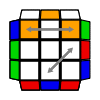U R U' L U L' U R' U' R B2 R B2 R2' R' F L F L' U2 R U R2 F R F' U F' R U' L' U R' U' R U' L U L' B2 L' B2 L2 y' R' F2 D R U' R' D' F R' F' R F' U R' F2 R2 y
D set
DAC DAI DAB DAMR' U R U' R' F' U' F R U R' F R' F' R U' R U2 F R U2 R' U y' R' F R' F' R2 U R' U' y y' F R U' R' U' R U R' F' R U R' U' R' F R F' y R2 U R U R' U' R2 F' U F R' F' U' F R2 U' R2
DAD DAE DBD DBE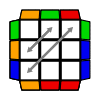R' U2 R U2 L U' R' U L' U L U' R U L' y2 R U2 R' F R2 F' U2 R2 F R' F' R2 U2 F R2 F' U2 y2 R U R' U R U R' F' R U R' U' R' F R2 U' R' U2 R U' R' R' F' U2 F U' R U R2 F R F' U2 R U
I set
IAC IAI IAB IAM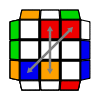y B' R U R' U' R' F R2 U' R' U' R U R' F' B y' (?) y R' F' U F R U R2 F R F' R U' y' y' U' R' U' R U R' F' R U R' U' R' F R2 y L' r' U2 R U2 R' F R U R' U' R' F' R2 U' M' L (?)
IAD IAE IBD IBEF R U R2 F R F' R U' R' F' U R' U2 R U R' U R F' L' U' L U F U y2 F R U R' U' R' F R2 U' R' U' R U R' F2 y2 y' F R U' R' F' U' F2 R' F' R F' U y
IBC IBI ICD ICE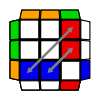L' R' U2 R U2 R' F R U R' U' R' F' R2 U' L l' y R U R' F' R U R' U' R' F R2 U' R' U' y' l (?) y' R' U2 R L U' R' U L' U2 R U' y y' R' U' F' R2 U y F R' F R U2 L U R' F' R

Algs from:
RobertY, Kirjava, bobthegiraffemonkey, acube, others

Images made using Visualcube